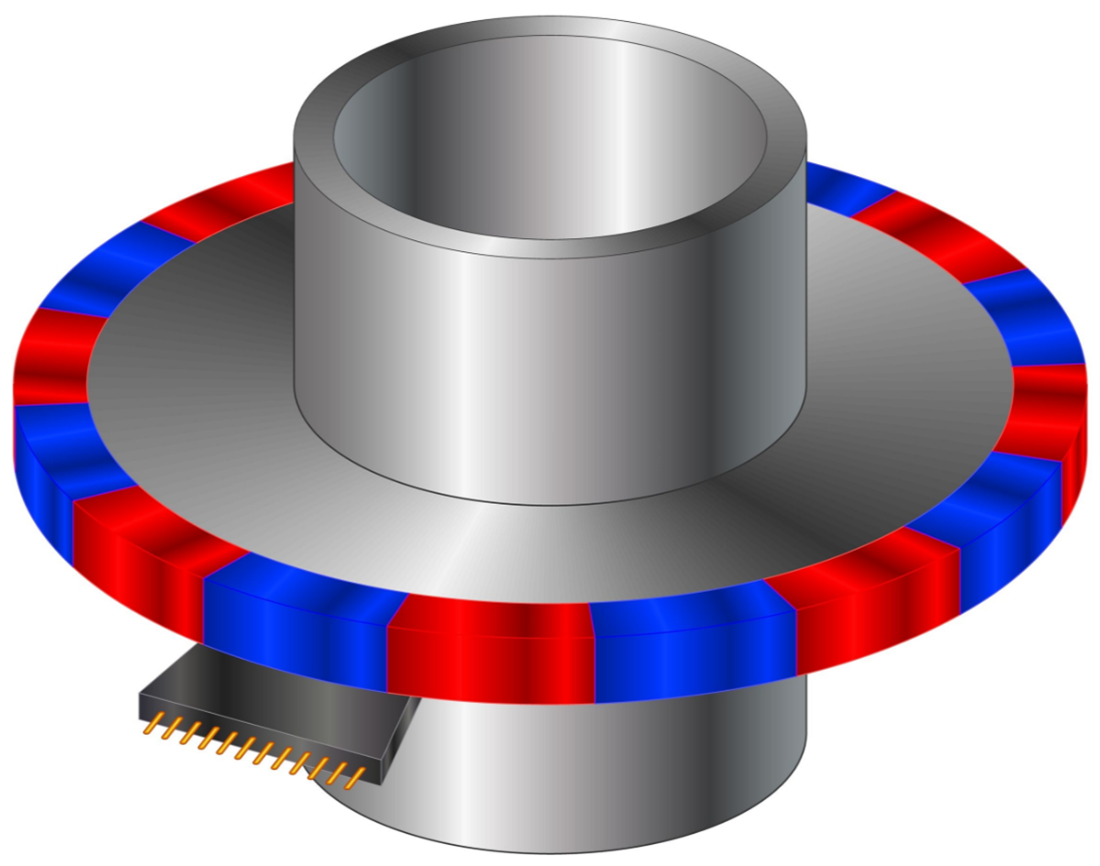Figure 1. Commutation sensor. Source: Shutterstock

# Design requirements

• 15 firing positions — No bounce
• Minimal hysteresiwe435s — 0° ideal (which is not possible)
• Speed functionality — Minimum speed 50 RPM when operating, otherwise 0
• Poor axial stack-up conditions — Alignment 0.035 inches axially from nominal position
• Good radial stack-up conditions — Radial position of sensor held to 0.005 inches
• Gap to be large as possible to allow for maximum air flow for cooling of motor (this counters the minimal hysteresis statement)
• Radial gap of motor is centered on a 2 inch diameter
• Radial gap of motor is held to 0.010 inch nominal to allow for concentricity issues as well as thermal expansion
• Lowest cost possible (also counters the minimal hysteresis statement)
• Due to electronics packaging, the magnet with sensor must be less than 1.5 inches in diameter.
• Temperature range of -40° C to 125° C, with storage temp of 150° C
1. Determine the types of Hall sensors that could work.
1. Switch — Will work as it provides the on-off digital output electronics that it will work with
2. Latch — This will also work but will require alternating poles
3. Linear sensor — This will not work without additional electronics
4. Power — This latching device will work, but the power capacity will be more expensive
5. Programmable — Switching device will work, but will have to program each chip, which is more expensive than conventional switch or latch
2. Analyze the options and select a sensor type.
1. Switches and latches are both valid and similar in cost
2. Power is not needed so no reason to use
3. Programmable will work and allows options should they be needed considering the cost increase
4. Based on the firing count of 15, a latching sensor with “reasonable” flux density switching points (Bop and Brp) would work well. Typically, the latching sensors will allow slightly lower to very reduced levels of required flux density and will help to minimize the hysteresis of the system.
3. Select a specific sensor to start the preliminary analysis.
1. Utilizing a Hall effect summary sheet from the manufacturer, select a sensor that meets the “reasonable” flux density level desired. Typically, this should be as low as possible to meet the hysteresis requirements, but not too low as there is a price versus performance trade-off.
2. In this case, selecting the 3281 L-UA from Allegro will allow the “middle” ground to be analyzed on a preliminary basis. Once the preliminary analysis is completed, the selection of the Hall sensor may be reviewed and adjusted as necessary.
4. Determine preliminary magnetic requirements.
1. Because this is a latch, the Hall sensor must see alternating north and south poles. The south poles will turn the sensor on and north will turn it off. This requires 15 pole pairs to receive 15 firing positions assuming that the system will only recognize leading edges of the electrical signal (when the signal turns on, not when it turns off). Pole pairs x 2 = total number of poles = 30 poles for this example.
2. Since air gap will be critical in minimizing performance variations in production, it is best to try and maintain this as consistently as possible. Because stack up tolerances are less in the radial direction (0.005 inches) then in the axial direction (0.035 inches), the sensor system will be the most consistent if the sensor is used in a radial fashion.
3. Because the sensor has an overall maximum thickness of 0.062 inches and a maximum width of 0.164 inches, the outside diameter of the air gap can be calculated. The flat of the sensor must fit inside the allowable diameter, so the radial position must first be calculated. This may be done with the following equation: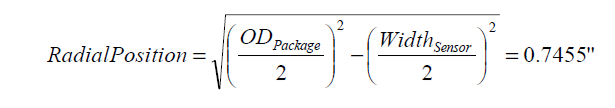Figure 2. Hall effect sensor, radial position equation. Source: Arnold Magnetic Technologies
The gap OR may then be determined by subtracting the sensor thickness from the radial position. This results in 0.6835 inches for a gap OR.
4. Applying the radial positional tolerance variation of 0.010 inches (0.005 inches) to the gap OR of 0.6835 inches yields 0.6735 inches as a minimum gap OR. Assuming that the motor clearance (radial gap) of 0.010 inches is on the tight side, adjust the physical air gap to a minimum of 0.015 inches for a safety factor. This results in a maximum magnet OR of 0.6735 inches – 0.015 inches = 0.6585 inches or a 1.317 inch diameter.
5. Using the magnet OD of 1.317 inches and applying a common tolerance rule, which is the greater of 0.3% or 0.002 inches, a tolerance of 0.004 inches may be assumed. This results in a nominal magnet diameter of 1.313 inches and 1.309 inches as a minimum diameter. The nominal arc length of a single pole may then be calculated as πD/N, where N is the total number of poles and D is the magnet OD. In this case, the arc length of a single pole is 0.1375 inches.
6. The total effective air gap (TEAG) is a key element in the design of Hall sensor applications. The TEAG is the distance from the surface of the magnet to the location of the Hall element located inside the Hall sensor. In this particular case, the minimum, nominal and maximum TEAG’s should be determined for nominal operating conditions as well as worst case analysis. For the Allegro 3281 L-UA package the Hall element is recessed inside the IC package, which is 0.0195 inches, and applying a typical tolerance of 0.003 inches results in a range of 0.0165 inches to 0.0225 inches recess.
7. The nominal TEAG may be calculated by the following equation: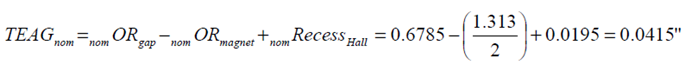Figure 3. Hall effect sensor, nominal TEAG calculation. Source: Arnold Magnetic Technologies
8. Calculate the maximum TEAG as follows: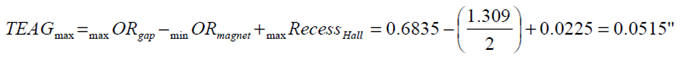Figure 4. Hall effect sensor, maximum TEAG equation. Source: Arnold Magnetic Technologies
9. Continuing on to calculate the minimum TEAG: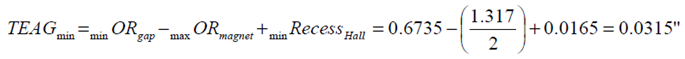Figure 5. Hall effect sensor, minimum TEAD equation. Source: Arnold Magnetic Technologies
10. Comparing TEAG to the basic gap equation of: TEAG WP / 2 where Wp is the arc length of a single pole. Wp is established in section <e> to be 0.1375 inches. This means that the TEAG 0.1375 / 2 = 0.06875 inches and the TEAG calculated in section <h> meets this criteria.
11. Determine the required axial length of the magnet based on the equation:
AL 2 TEAG Tap where Tap is the tolerance of axial position alignment. Tap must include the positional tolerance of the IC and the positional tolerance of the Hall element internal to the IC. In this example, AL = 2 x 0.0515 inches + 0.070 inches + 0.010 inches = 0.183 inches for the preliminary analysis.
12. The only dimension of the magnet not identified at this point is the ID of the magnet. In order to provide a starting point for the ID of the magnet, some generic assumptions must be made. Assuming that an operating PC 1 is acceptable, one may estimate the ID of the magnet per the following equations: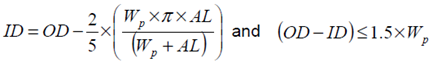Figure 6. Hall effect sensor, inner diameter equation. Source: Arnold Magnetic Technologies
where Wp is the arc length of a single pole, OD and ID refer to the outer and inner diameters of the magnet and AL refers to the axial length of the magnet. The first equation is only useful for obtaining a starting point when experience does not provide a guideline.
The second equation should usually be a constraint that the magnet must adhere to because it carries a relationship involving successful magnetization as well as a point of diminishing returns to the volume of the magnet. While segments may be pre- magnetized and glued together to obtain parts outside of this spectrum, it is not necessarily the best way to solve many designs. If molding a magnet to size, it is necessary to model with this limitation in place as magnetization will not significantly penetrate beyond this condition. Any additional gains will just provide additional safety factors to the design.
Based on the first equation, the ID may be estimated as 1.214 inches and the second equation validated by 0.099 < 1.5 x Wp = 0.206 inches. Adjustments during the refining of the design may occur but should still fit the second equation.
5. Analyze the preliminary design.
1. Analysis of the design requires selecting a material to use in the application. Based on the temperature and the multi-pole on the OD design, either sintered ceramic 1 or a radially oriented bonded ferrite magnet is likely to provide the most cost-effective solution. Due to the tolerance used of 0.3% the ceramic would require final grinding or a bonded magnet molded to size. In this example, the design will be based around a bonded ferrite magnet that is radially oriented. For this part’s temperature requirements and no stress condition, Plastiform 2060 will make an excellent low cost option.
Using an FEA/BEA or other custom package, analyze the flux density condition around the magnet based on nominal dimensions and tolerances. The best way to look at this initial information is a flux density versus rotation plot as shown below. Note there are only two poles shown as the remaining poles will theoretically have the same performance.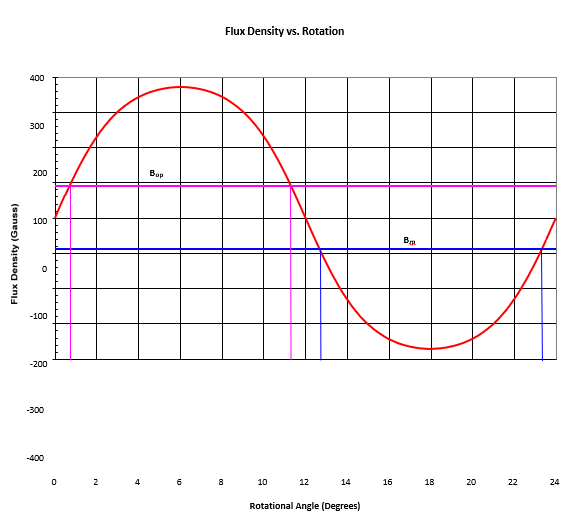Figure 7. Flux density vs. rotation. Source: Arnold Magnetic Technologies
2. Plot out the max Bop and min Brp values to determine the rotational hysteresis from the system. The graph shows that the rotational hysteresis will be about 1.5°.
3. Determine if this design is in the acceptable range. If lower hysteresis is desired, then either convert to a material that will provide a higher peak flux density, which in turn will create a faster transition rate and lower rotational hysteresis or identify another sensor that will switch at lower levels.
If the hysteresis is tighter than required, consider a chip with higher switching levels or a weaker material. In this case, a non-oriented (isotropic) material might be acceptable.
For this example, an acceptable level of 3° hysteresis will be assumed, which maintains a little safety factor in this design to cover the temperature and tolerance aspects.
6. Begin worst case analysis of design.
1. Using worst case Pc conditions, analyze the magnet for the operating load line. Worst case Pc for a ring magnet magnetized on the OD occurs with the following magnet dimensions:
· ODmin = 0.654 inches
· IDmax = 0.609 inches
· ALmax = 0.186 inches
By using a 3D FEA / BEA system or other custom software, the load line of the magnet may be found by determining the Bdave and Hdave at the neutral zone. The formula (Pc=Bd/Hd) may then be applied with these average values. This results in a Pc = -1.27 for this ring magnet condition.
2. Using the Pc value calculated, as well as the demag curve for this material, calculate the expected irreversible losses to this system. This requires use of the intrinsic permeance coefficient or Pci = Pc + 1 when using the absolute values for Pci and Pc. In this case, Pci = 2.27 and is the slope plotted on the demagnetization curve.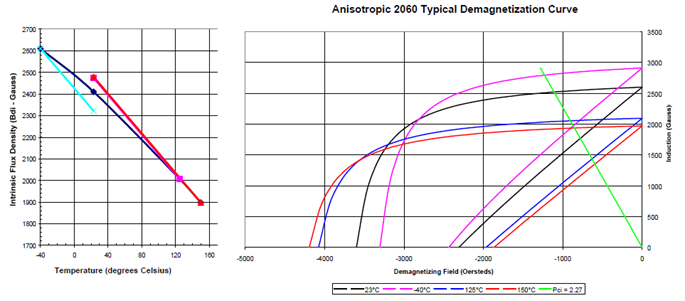Figure 8. Anisotropic 2060 typical demagnetization curve. Source: Arnold Magnetic Technologies
Analyzing the intrinsic demagnetization curve and finding the intersections of each temperature curve with the intrinsic permeance coefficient will yield the temperature intrinsic induction (Bdi) values. Plotting these values versus temperature will show the performance as the temperature changes. Applying the reversible temperature coefficient to these temperature Bdi values will result in calculation of the short-term irreversible loss associated with this permeance condition. In this case, the STILT irreversible loss for -40° C will be 3.7% and you will note that the positive temperatures result in an increase in Br. This is not something that will happen as it will actually follow the original curve in this case. Using the value of 3.7% and doubling to simulate the long term irreversible losses (LTILT) results in an expected decay of 7.4%.
3. Identify conditions that create the worst-case scenario for the design.
· TEAGmax condition
· Maximum offset of Hall sensor alignment
· Maximum operating temperature
· Thermal demag due to TcHci (occurs at coldest temperature in ferrite)
· Least material condition of magnet (LMC), both physically and magnetically
4. Re-analyze system using these worst-case conditions:
· TEAGmax = 0.0515 inches
· ODmin = 0.654 inches
· IDmax = 0.609 inches
· ALmin = 0.180 inches
· Gap Radius = 0.0515 + 0.654 = 0.7055 inches
· Axial offset of sensor = 0.040 inches
· Brmin at 125° C = 2,090 – 7.4% irreversible loss = 1935 Gauss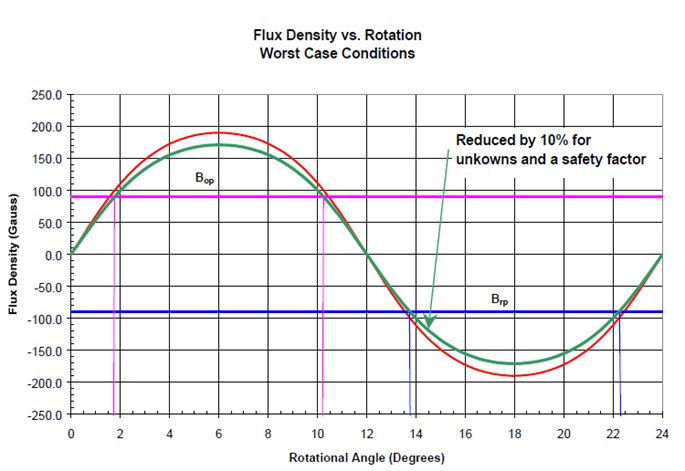Figure 9: Flux density versus rotation. Source: Arnold Magnetic Technologies
· Hcmin at 125° C = 1,845 adjusted by 1845/2090 *1935 = 1708 Oe
Applying a 10% reduction to the rotational curve helps to serve as a safety factor in the design and for the potential of having missed some additional criteria. Once the flux density versus rotation curves are generated, the Bop and Brp values should be analyzed to determine the worst case rotational hysteresis. In this case, the rotational hysteresis has increased to 3.5° and is outside the assumed acceptable limit of 3°. At this point, the design needs to be reviewed and determine if 3.5° is acceptable or if the design needs to be modified to reduce the hysteresis to less than 3°. If a design modification is required then reviewing stronger magnetic materials and Hall sensors with lower Bop and Brp would be two common approaches to resolve this issue as well as adjusting the TEAG (less common as not always controllable). The assumption that the rotational hysteresis may now be up to 4° will be used in this example.
7. Determine the critical evaluation factors for the design. They are usually contradictory in nature, so they will have to be weighted appropriately. Some example factors are:
· System cost
· Solved by reducing AL and increasing ID to meet specifications and minimize magnet volume.
· Minimize irreversible losses
· Solved by reducing AL and decreasing ID to maximize length to area ratio or changing materials.
· Minimize variation in magnetic performance versus positional tolerances
· Solved by increasing AL of magnet to improve axial offset results. This may also result in the need to decrease the ID to maintain an acceptable Pc condition and irreversible loss value.

The potential solutions to these various factors demonstrate the contradictory nature in optimization. Balancing these factors to an acceptable solution is often a difficult and time-consuming process.

# Design checklist

1. Start the “generic” design by selecting appropriate type of Hall sensor
· Switch — Digital output
· Latch — Digital output requires alternating poles
· Linear sensor — Analog output proportional to B
· Power — High current latching device
· Programmable — Switching or linear with adjustable properties
2. Review sensors of desired type and select a “starting” point
3. Determine preliminary magnetic requirements
· Pole configuration
· Alignment concerns
· Restricted space limitations
· TEAG variation
· Initial size of magnet
4. Analyze the preliminary design
1. Select an initial material
2. Use magnetics software such as BEA/FEA or custom package to analyze output of nominal conditions
3. Analyze output of magnet with respect to Hall sensor specifications
1. Could be linearity, Bop, Brp, Bhys, physical hysteresis
2. Determine if response is in acceptable range
1. If not, then re-choose sensor or magnet material or configuration and begin the process again
2. Once satisfied, proceed to step 5
5. Begin worst case analysis of design.
1. Calculate Pc for worst case permeance
2. Use the Pc calculated along with the intrinsic demag curve to predict irreversible losses.
3. Identify worst case scenario for the sensor systems design
4. Analyze system for worst case scenario including irreversible losses
1. If system fails requirements, return to factors under design control and start again
2. If system passes requirements, continue on to step 6. Some designs may require comparison to best case conditions or opposite spectrum conditions for complete variation analysis
6. Evaluate design for “critical factors” determining final design variation.
1. System cost
2. Minimize irreversible losses
3. Minimize variation in magnetic performance versus positional tolerances
7. Repeat all steps above as necessary to finalize design.

# Checklist

Select type of Hall effect device

▢ Switch
▢ Latch
▢ Linear
▢ Power
▢ Programmable Switch
▢ Linear
Select Hall effect device Manufacturer:
Model number: __________________

Determine preliminary magnetic requirements

▢ Pole config.
▢ Alignment tolerances
▢ Space limits
▢ Initial magnet size
▢ TEAG
▢ Min
▢ Nominal
▢ Max
Temperature range: __________________
Select material: __________________

Analyze magnetic output

▢ Bop
▢ Bhys
▢ Linearity
▢ Brp
▢ Physical hysteresis
▢ Saturation of Hall
Calculate worst case Pc: __________________
Predict irreversible losses: __________________

Determine worst case operating conditions

▢ TEAG max
▢ Max offset position
▢ Irreversible losses
▢ Temp max
▢ LMC magnet
▢ Min magnet props.

Apply safety factor to magnetics:

▢ 5%
▢ 10%
▢ 15%

Perform final analysis of design

▢ Bop
▢ Bhys
▢ Linearity
▢ Brp
▢ Physical hysteresis
▢ Saturation of Hall

Optimize design for critical factors

▢ Price
▢ Irreversible losses
▢ Variation reduction
▢ Other: __________________